Dr. J's Maths.com
Where the techniques of Maths
are explained in simple terms.

Trigonometry - Trigonometric identities.
Test Yourself 2 - Solutions.

Simplify the following trigonometric identities:

 Basic substitutions. 1. 2. 3. 4.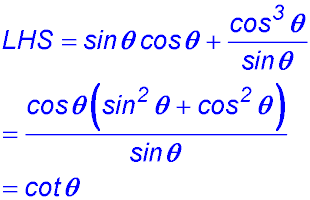Complementary angles. 9.10.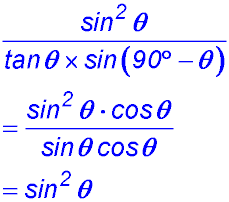Pythagorean relationships. 23. 24. 25. 26.

Prove the following trigonometric identities:

 Basic substitutions (change of definition). 15. 17. 19. 21. Pythagorean relationships. 23.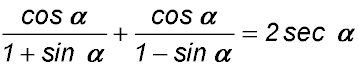24.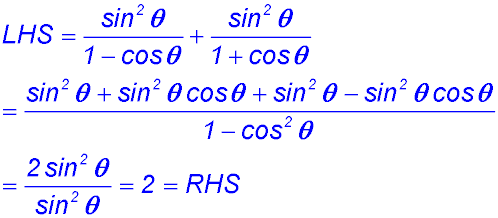tan x - tan x sin2 x = sin x cos x 2 sec2α - 3 = 2 tan2α - 1 25.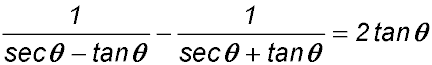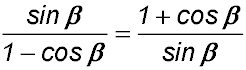Mixed relationships.